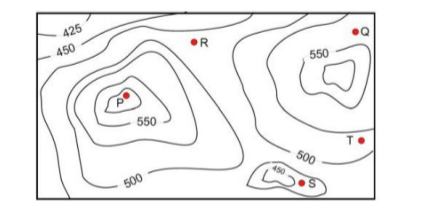# GATE | GATE-CS-2017 (Set 1) | Question 9

A contour line joins locations having the same height above the mean sea level. The following is a contour plot of a geographical region. Contour lines are shown at 25 m intervals in this plot.If in a flood, the water level rises to 525 m, which of the villages P, Q, R, S, T get submerged?
(A) P,Q
(B) P,Q,T
(C) R,S,T
(D) Q,R,S

Explanation: Given data – height of all villages

• P = 575
• Q = 525
• R = 475
• S = 425
• T = 500

Villages  below the flood level – 525m are

1. R <500m
2. 425m<= S<=450m
3. 500m <=T<= 525m

Therefore option C is correct.

Quiz of this Question

My Personal Notes arrow_drop_up
Article Tags :

Be the First to upvote.

Please write to us at contribute@geeksforgeeks.org to report any issue with the above content.